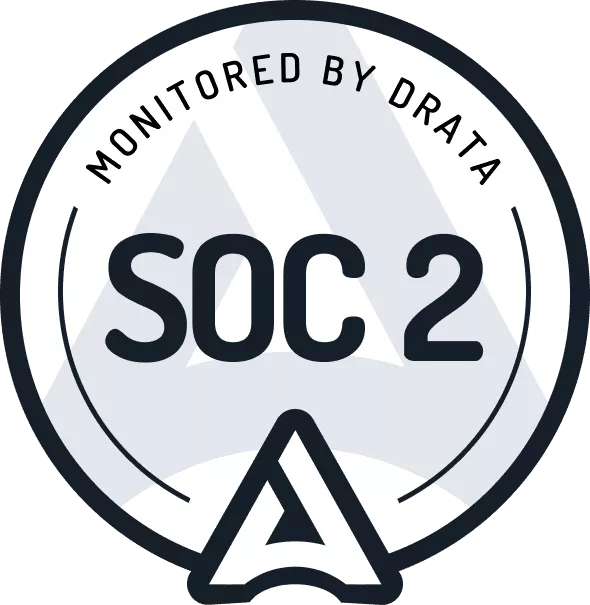# How to calculate a lease liability and right-of-use asset under IFRS 16

2021-07-10 | Lucas Russell

## Under IFRS 16 brings new lease accounting requirements

When accounting in compliance with IFRS 16/AASB 16 as a lessee, the party leasing the asset, all leases in the scope of the standard must be recognized on the balance sheet. That's a significant change to the previous accounting under IAS 17/AASB 117. Under the last lease accounting model, the lessee first classified the lease as either an operating or finance lease. That classification had a significant impact on the accounting for the lease.

Under IAS 17, if the risk and rewards did not transfer to the lessee, the accounting was straightforward. The lease would be classified as an operating lease, and you would account for the lease expense when incurred.

If the risks and rewards transfer to the lessee, the lease would be classified as a finance lease under IAS 17. The lessee would be required to recognize a leased asset and liability. The accounting for a finance lease was far more time-consuming than that of an operating lease.

Under the previous lease accounting standard, given the nature of lease transactions, on most occasions, a lease would be classified as an operating lease instead of a finance lease.

That's all changed under IFRS 16 / AASB 16, as there is no need to determine the lease classification, the accounting for a lease is now consistent. However, for many, especially the person required to do the accounting, that's where the good news stops. Under IFRS 16, the accounting closely reflects the accounting for a finance lease under IAS 17 / AASB 117.

## Accounting for a lease under IFRS 16

Anyone familiar with finance leases under the previous lease accounting standard will understand that the required accounting is far more intensive. This is because every lease requires:

1. A net present value calculation is required for every lease, which represents the liability associated with the lease
2. The calculation of a leased asset which is based on the liability amount
3. Any changes to the future payments of the lease require an update to these calculations

Under the new lease accounting standard IFRS 16 / AASB 16, the net present value calculation is referred to as a lease liability, and the leased asset is referred to as the right of use asset.

This article will cover two practical examples of how to calculate for a lease as a lessee under IFRS 16. The first will focus on the initial recognition of the lease liability and right of use asset. In other words, how to calculate a lease liability and right of use asset if there are no subsequent changes to the lease. The second example will cover how to account for a lease modification, which is a change that occurs after the commencement of the lease. Example 2 will highlight how modifying the lease impacts the value of the lease liability and right of use asset.

This article assumes that you understand the principles of the time value of money and present value techniques. If you are unsure, I'd highly recommend reading this article:

That's because, under IFRS 16 / AASB 16, everything is based on the concept of the present valuing a set of future payments.

## Where to start - the lease liability

A lease agreement can be very long and convoluted. From a lease accounting perspective, three main inputs need to be extracted to be able to calculate the net present value calculation of the lease liability:

1. The commencement of the payments, aka the commencement date of the lease
2. The payments required over the lease life, aka the lease term
3. The end date of the lease payments also referred to as the expiry of the lease

These three inputs determine the future lease payments, which the lessee is required to present value.

The final input is the discount rate. The discount rate is the input used to calculate the present value of the known future lease payments. This figure is not extracted from the lease agreement.

Commencement date

The start date of the lease can be a judgment call. For further information on how to determine the commencement date of the lease, refer to:

Lease payments

With lease payments, there are three types of lease payments:

Lease payment type Description Include in lease liability calculation?
Fixed payments These payments are known at the inception of the lease. They are the payments made by a lessee to a lessor for the right to use an underlying asset during the lease term. This will typically consist of a regular payment based on a specific frequency such as monthly, quarterly, or annually. If a company were reasonably likely to exercise a purchase option within the contract which incurred a payment. You would include that payment as well. Yes
Variable lease payments Variable lease payments: these are payments that depend on an index or a rate, initially measured using the index or rate as at the commencement date. Common examples include the increase in fixed payments with the consumer price index (CPI) or market rent review (MRR) at a stipulated point in time. Yes
Truly variable lease payments Variable lease payments that depend on sales or usage of the underlying asset are excluded from the lease liability. Instead, these payments are recognized in profit or loss in the period in which they occur. No

Lease term

Like with the other two components, determining the length of the lease requires judgment. The lessee is to select the most reasonably likely end date of the lease. This may or may not include renewals.

For further information, refer to IFRS 16, paragraph 18.

Discount rate

The lease payments shall be discounted using the interest rate implicit in the lease if that rate can be readily determined. If that rate cannot be readily determined, the lessee shall use the lessee’s incremental borrowing rate. This is a judgment call, and you should be able to justify the discount rate being used. For more details refer here.

## Right of use asset

The majority of the value attributed to the right of use asset amount is derived from the lease liability value. When initially recognizing the value of the right of use asset, the other inputs that impact the value are:

• The make good provision: any costs that will be incurred restoring the leased asset to a necessary condition
• Any direct costs incurred during the initiation of the lease
• Any lease payments made at or before the commencement date, less any lease incentives receivedFor further details, refer to IFRS 16, paragraph 24.

Once you have determined these values, the time has come to calculate the lease liability and right of use asset in accordance with IFRS 16 / AASB 16.

## Modification accounting

Before going any further, it's essential to highlight modification accounting. So firstly, what is modification accounting? That's when there is a change to one of the inputs that determine the calculation of the lease liability. This will either be:

• Lease payments
• Lease term

A change to the lease calculation inputs can be a result of either:

a) A modification to the contract's terms and conditions or;

b) The lessee decides to exercise an option within the contract, for example, a renewal option.

When either scenario occurs, the lessee must recalculate the lease liability and, in turn, the right of use asset. As a result, modification accounting can get quite tricky, especially with a decrease in scope calculation. The devil is in the detail. For more information on this, refer to our IFRS 16 guide

Example 2 demonstrates how to account for the lease liability and right of use asset when there is a lease modification.

## Practical examples

These examples will take you through from scratch how to calculate the lease liability and the right of use asset. To be able to follow the examples, there are some prerequisites.

• You understand the time value of money and how to calculate the present value. If you’re unsure of this, refer here.
• You understand when entering a lease, the lessee recognizes a lease liability and right of use asset.
• The lease liability will then unwind to zero upon completion of the lease, as there are no more lease payments.
• The right of use asset will be depreciated to zero based on the useful life of the leased asset. This may or may not be the same date as the accounting lease end date.

The calculation methodology we will use to calculate the lease liability is the XNPV function within excel. This present value function requires to inputs:

• The date of the payment
• The amount of the payment

Because lease payments can occur on an ad hoc basis, calculations will be performed daily. This is to ensure ultimate flexibility, especially if a lease modification occurs. In addition, the upfront time investment is comparable to performing a monthly calculation which will not nearly be as accurate or as flexible as a daily calculation.

If you would like a copy of the calculation, reach out to [email protected]. Following along with the calculation will make it a lot easier to grasp the concepts and use them as a template for your lease calculations.

So now you’re ready to calculate the lease liability and right of use asset.

## Example 1 Scenario

• Commencement date: 2021-01-01
• Fixed payment amount: \$1,000
• Payment frequency: Monthly
• Accounting end date: 2021-12-31
• Discount rate: 7%
• CPI Increase at 2021-06-15

### Step 1 - work out the known future lease payment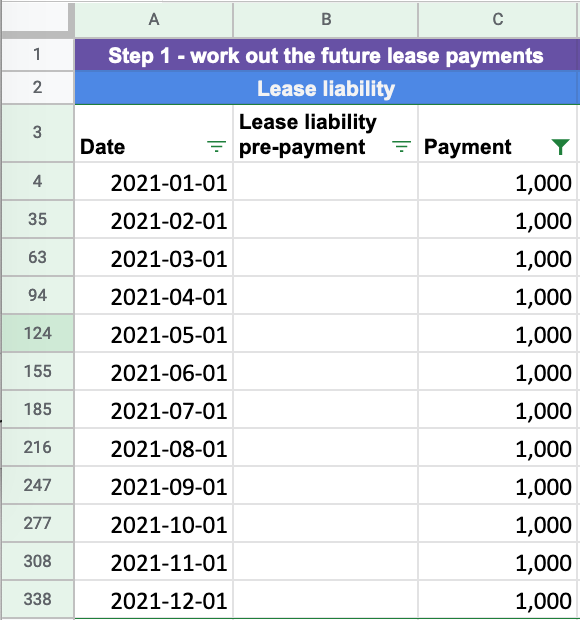These are the future payments related to the lease. Once these payments are present valued, it forms the lease liability. In this example, there are 12 payments of \$1,000 paid at the start of each month.

Example 1 also includes a CPI increase. However, at the lease commencement, the CPI increase has no bearing on future lease payments. That's because the lessee does not know at the beginning of the lease (2021-01-01) what the CPI increase will be.

If you want further information on what future payments will be used when determining the lease liability, refer here

### Step 2 - Apply the discount rate and calculate the lease liability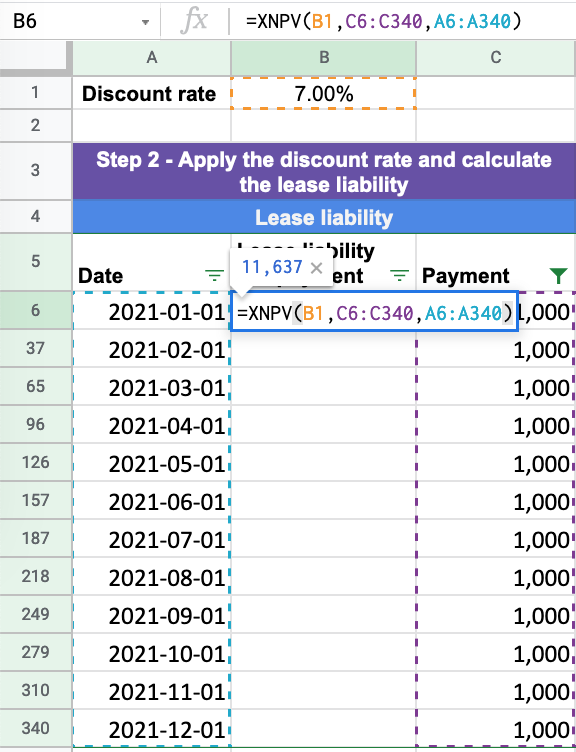The discount rate is not extracted from the details included in the lease agreement. Instead, it's a judgment call by the lessee. For further information on how to determine the appropriate discount rate refer here

In step one, we collated the future lease payments and the date of those payments. With the discount rate, we have all the necessary inputs to perform the present value calculation.

In this example, we use the XNPV function in excel because that's the most accurate present value calculation. If you're curious how the XNPV calculation is different from the NPV or PV function in excel, refer here.

For Example 1, the inputs are:

• Rate: 7%
• Values: twelve \$1,000 payments at the start of each month
• Dates: 2021-01-01 to 2021-12-31

From inputting the above into the XNPV formula, it results in the present value calculation of \$11,637. This is the lease liability balance at the commencement of the lease.

### Step 3 - Calculate the right of use asset balance

The right of use asset starts from the lease liability balance. Then several inputs can impact the value. For those inputs, refer here

Concerning Example 1, no other inputs are applicable. As a result, the right of use asset value matches the lease liability of \$11,637.

### Step 4 - Calculate the unwinding of the lease liability to zero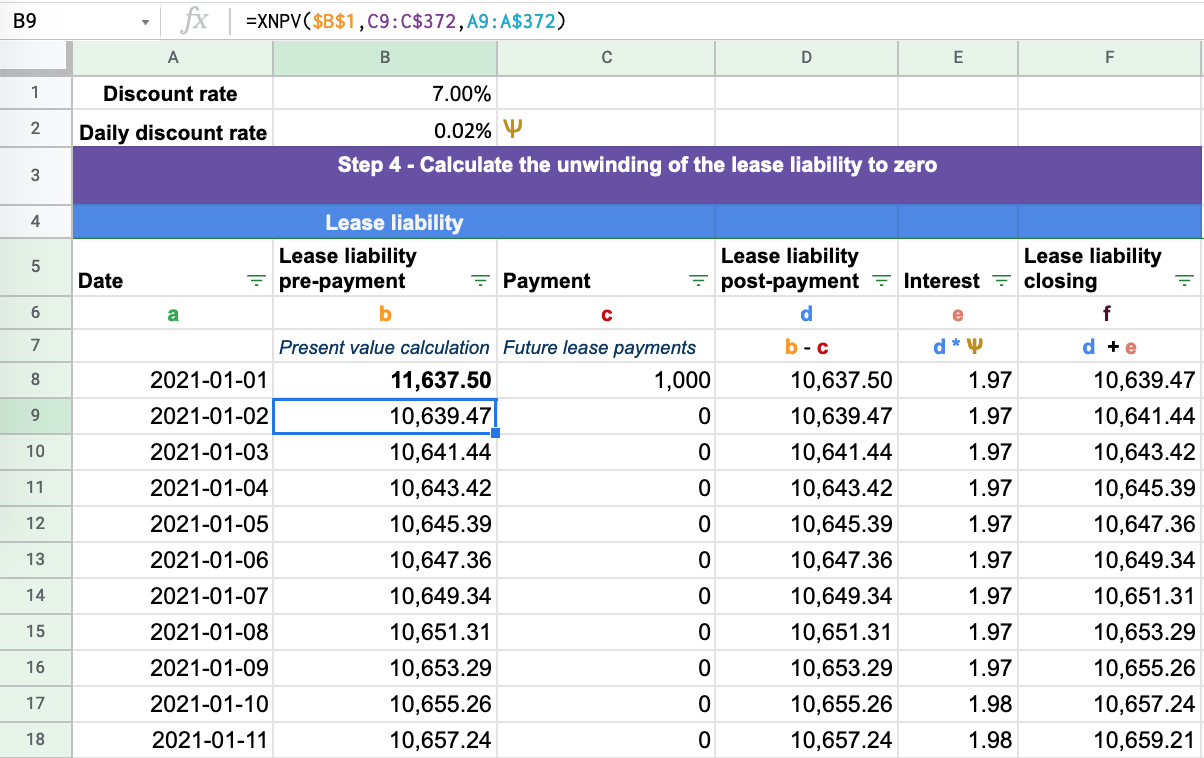From the present value at the commencement of the lease of \$11,637, we have all the required inputs to unwind the lease liability to zero. The lease liability should always unwind to zero upon the expiry of the lease. If the lease liability does not, it’s a simple check that there has been an error in the calculations.

So now, we will break down the formulas input in each column.

Column A - Date - This captures each day within the agreement. In this example, the commencement date is 2021-01-01 to the lease expiry date 2021-12-31: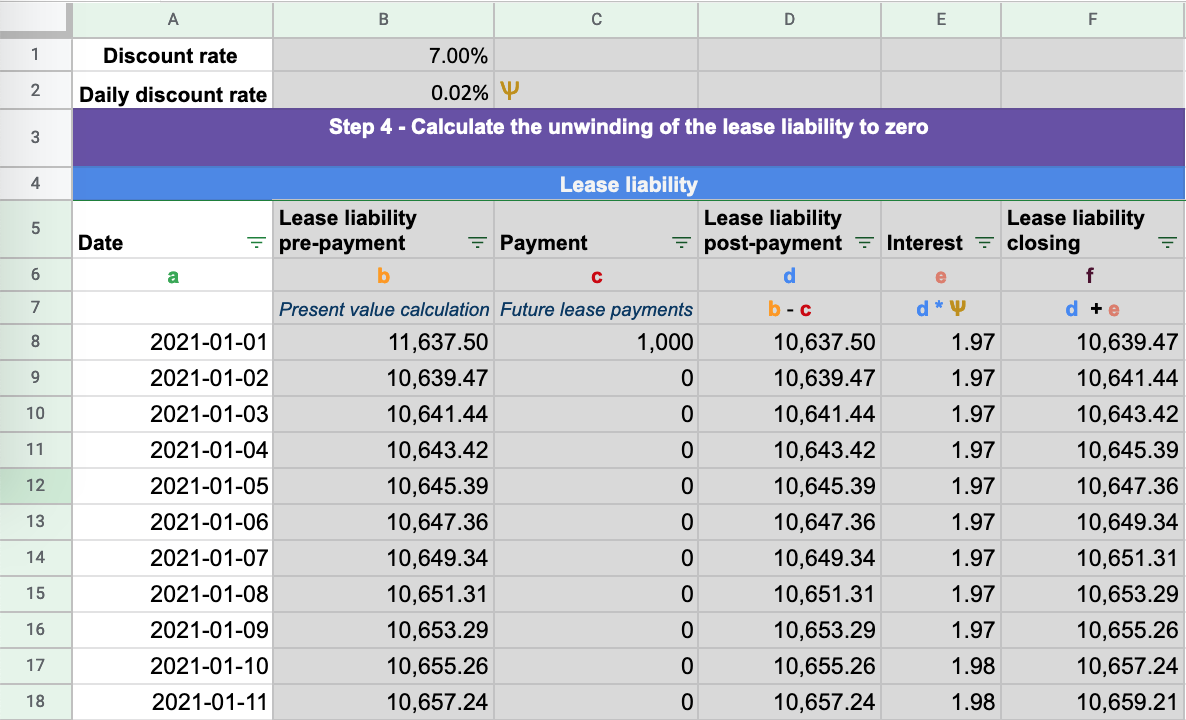Column B - Lease liability prepayment - This is the column where you input the XNPV formula in each row. Note there are several inputs in the calculation that are fixed. These are the discount rate, the ending cash flow amount, and the end date.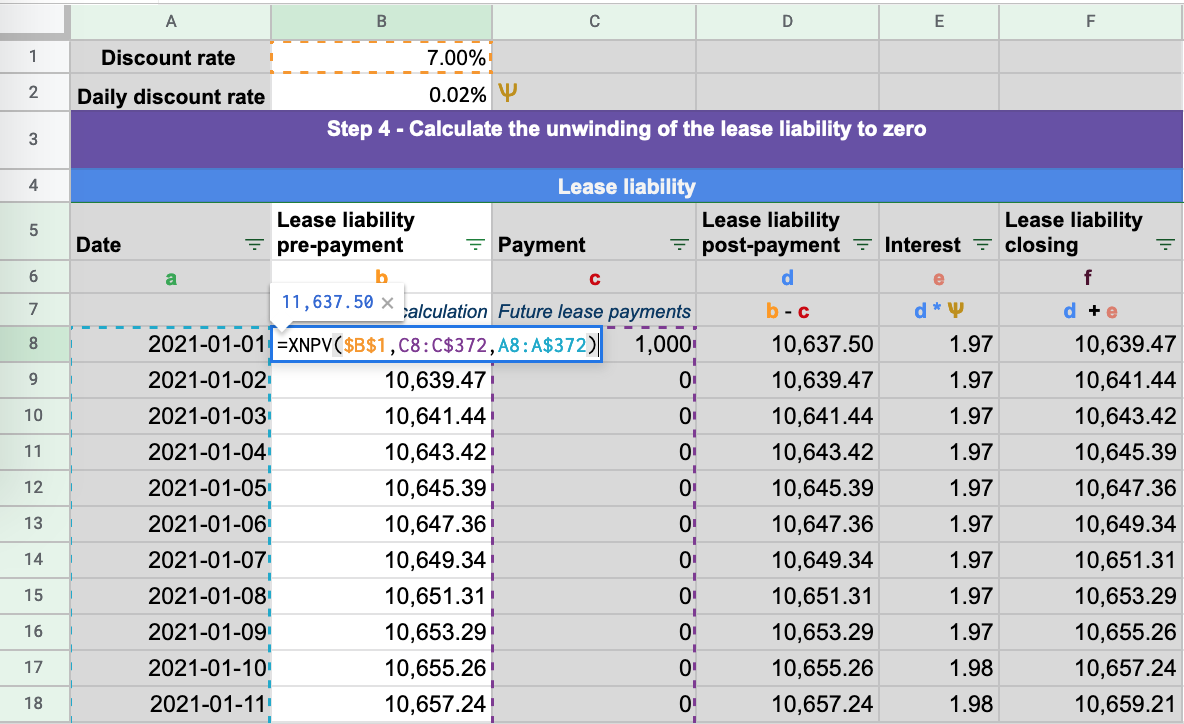Column C - Payment - The lease payments at each particular date: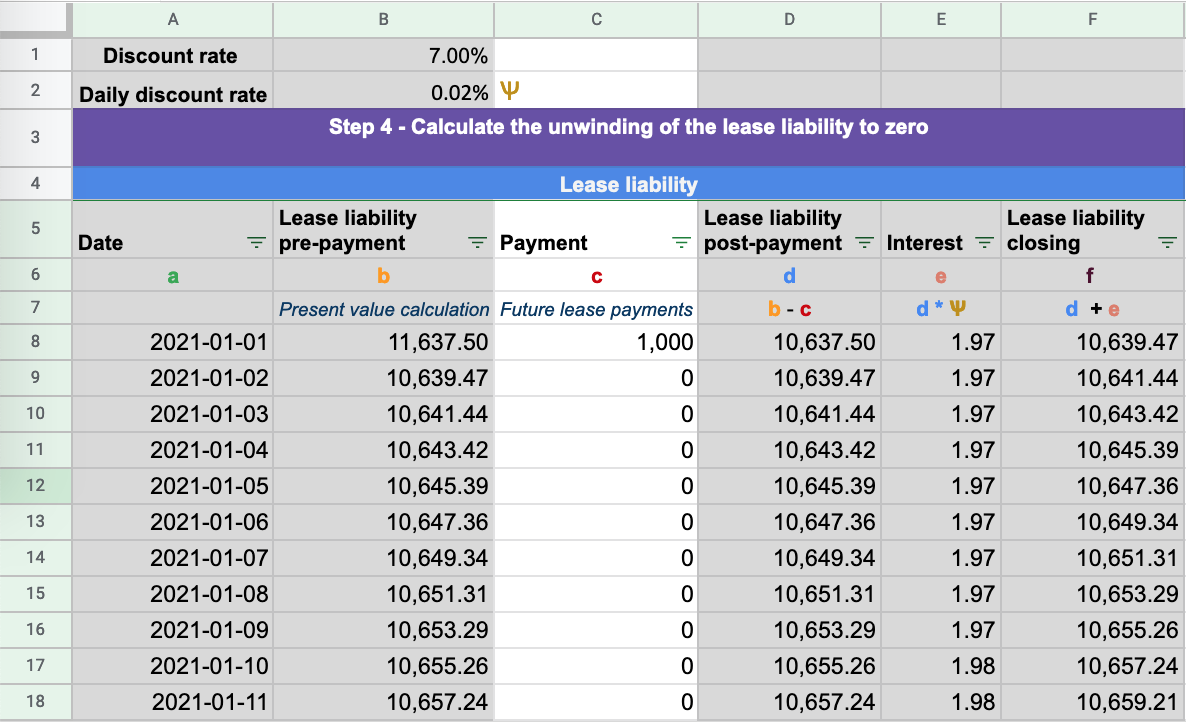Column D - Lease liability post-payment - This is the lease liability balance post payments. Each payment reduces the lease liability amount.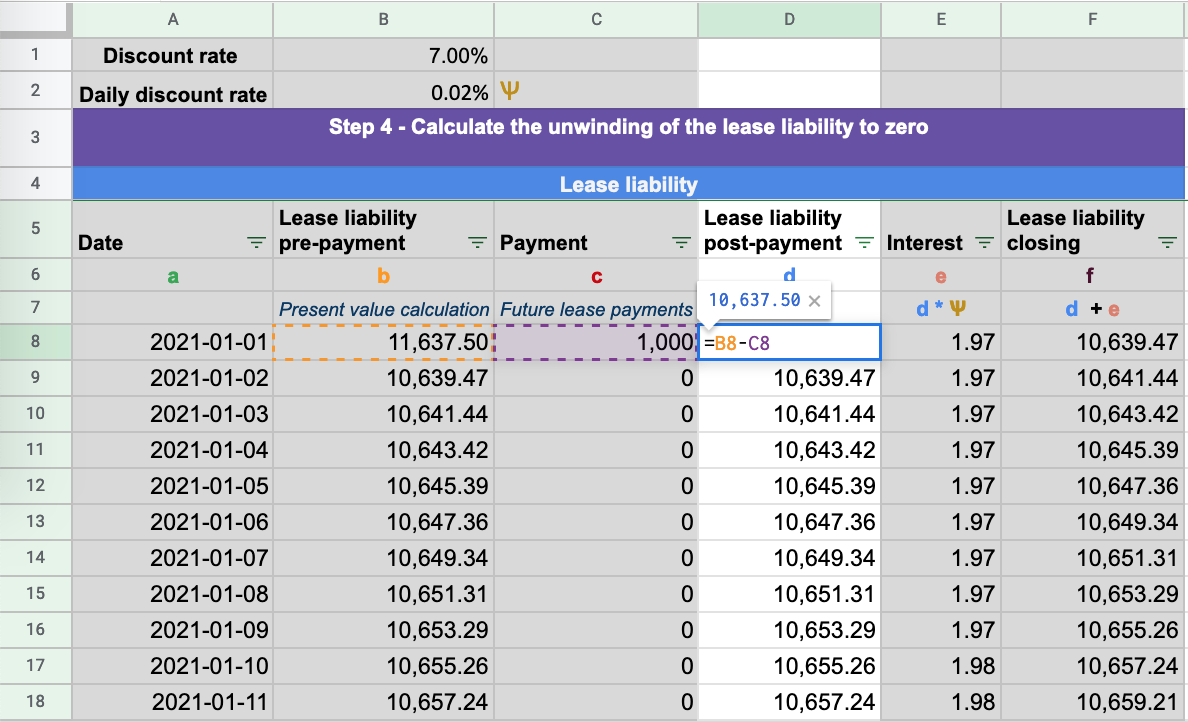Column E - Interest - This is the daily interest amount calculated on the lease liability based on the daily discount rate: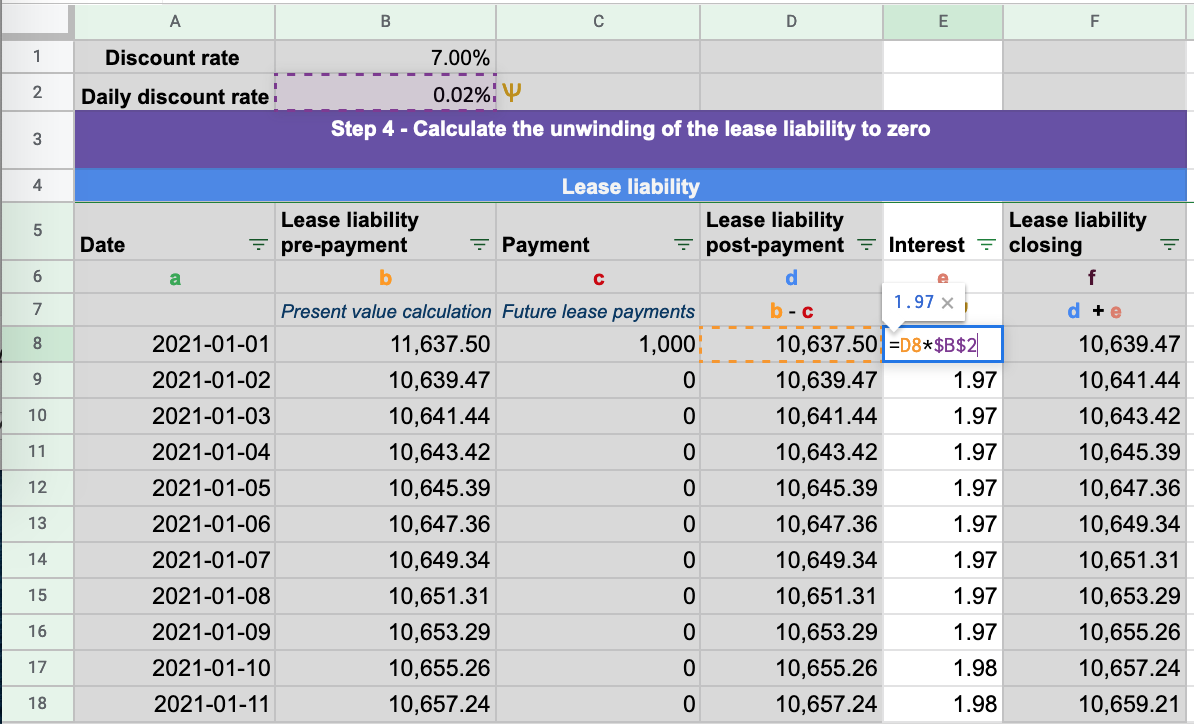To formula used to calculate the daily discount rate is the following:  (1+discount rate)^(1/365)-1

Column F - Lease liability closing - This is the closing balance of the lease liability for the day which includes the interest incurred. This value is the opening balance for the next day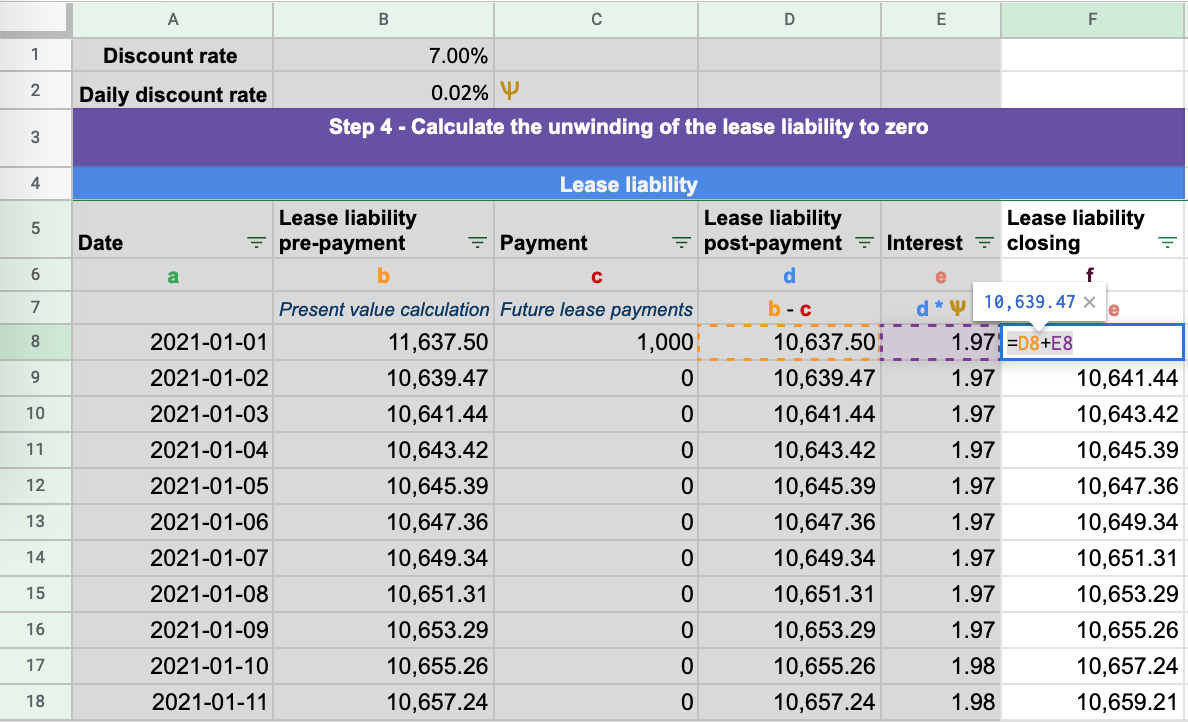The final test is to ensure the lease liability unwinds to zero: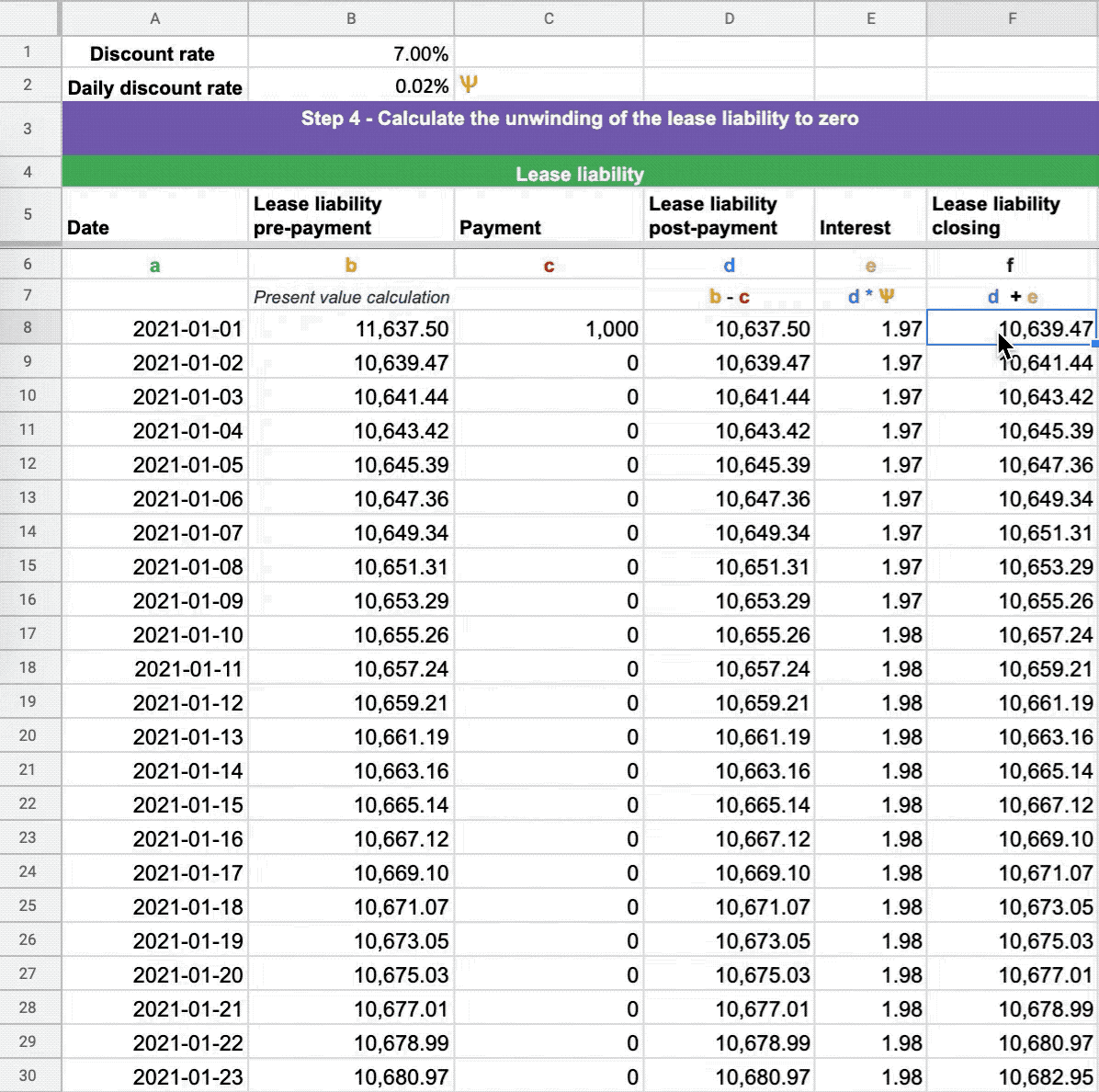## Step 5 - Calculate the depreciation of the right of use asset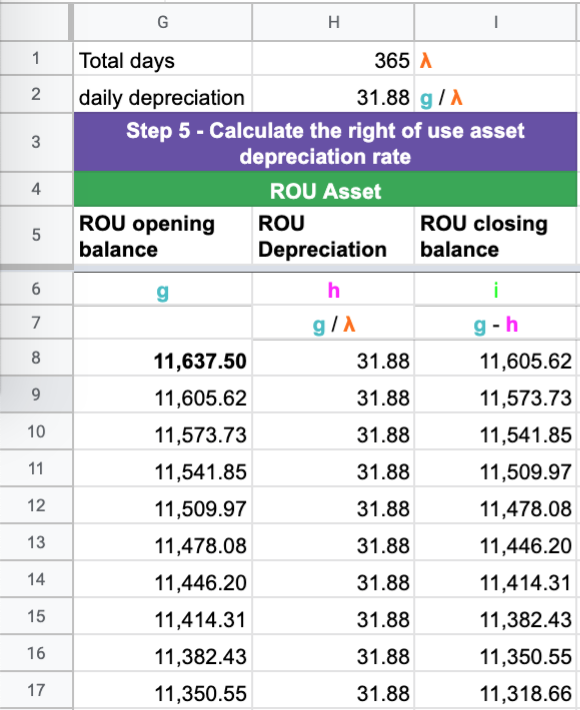Once the lease liability has been calculated, it's time to work out the depreciation of the right of use asset. The standard states a lessee can use any systematic method. The most straightforward approach is a straight-line calculation.

This calculation is the opening balance of the asset's right of use asset divided by total days in the lease. This then the daily depreciation rate.

Here are the steps to calculate this:

a) Calculate the opening balance of the right of use asset and divide by the total number of days the asset will be used. On most occasions, this will be the end date of the lease.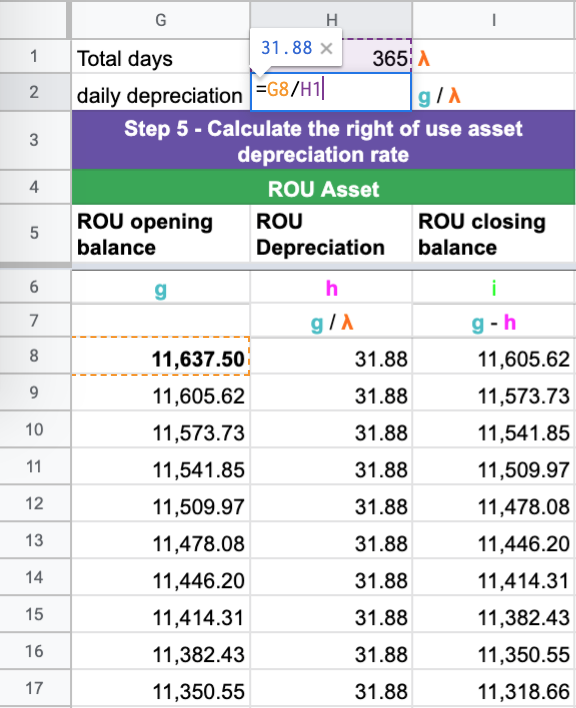b) Deduct the depreciation amount from the right of use asset amount for each day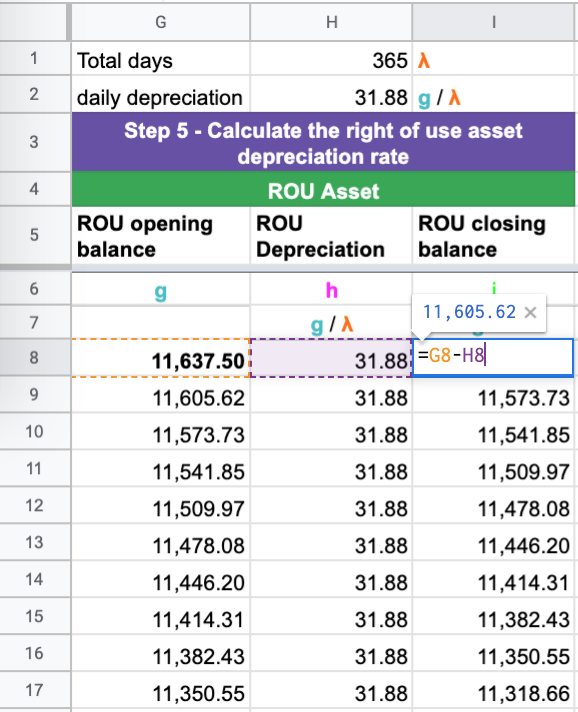c) Ensure the right of use asset depreciates to zero: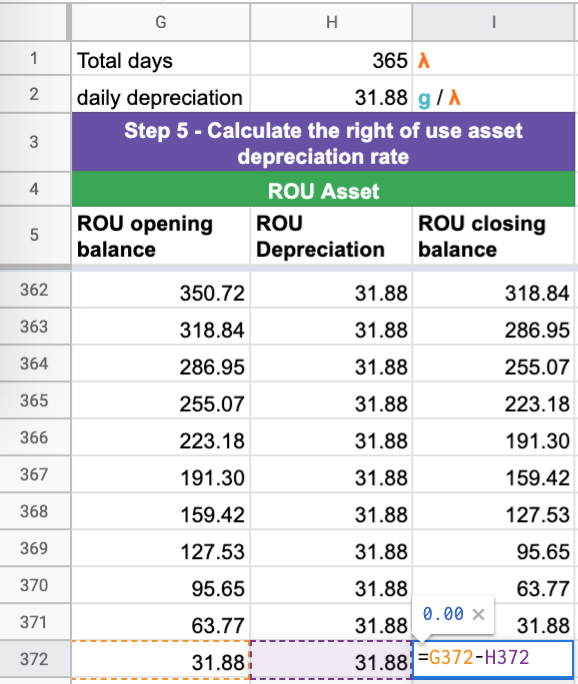With the assumption that there are no modifications to the lease, you have now calculated a lease in compliance with IFRS 16 / AASB 16.

## Example 2 Scenario - Modification accounting

In this example, we’ll cover how to account for a modification in a lease. A modification will result in changing either one or both of these inputs:

• lease payments
• Lease term

When a modification occurs, it results in the remeasurement of the lease liability based on the updated future lease payments. Generally, a modification is either an increase or decrease in scope. For a decrease in scope modification, which occurs either through a reduced lease term or reduction in the asset size, there’s an extra gain/loss calculation required. For more details on modification accounting, refer here

In Example 2, we will go through the steps involved when a CPI increase is communicated after the lease commencement.

Using the details of Example 1:

• Start date: 2021-1-1
• Accounting End date: 2021-12-31
• Fixed Payment Amount: \$1,000
• Payment Frequency: Monthly
• Discount rate: 7.00%

Modification to the terms:

• On 2021-06-15, the lessor communicated a CPI increase of 5% due for all payments from 2021-07-01
• The appropriate discount rate at modification is 6.00%

### Step 1 - Update the future lease payments from the date the modification is effective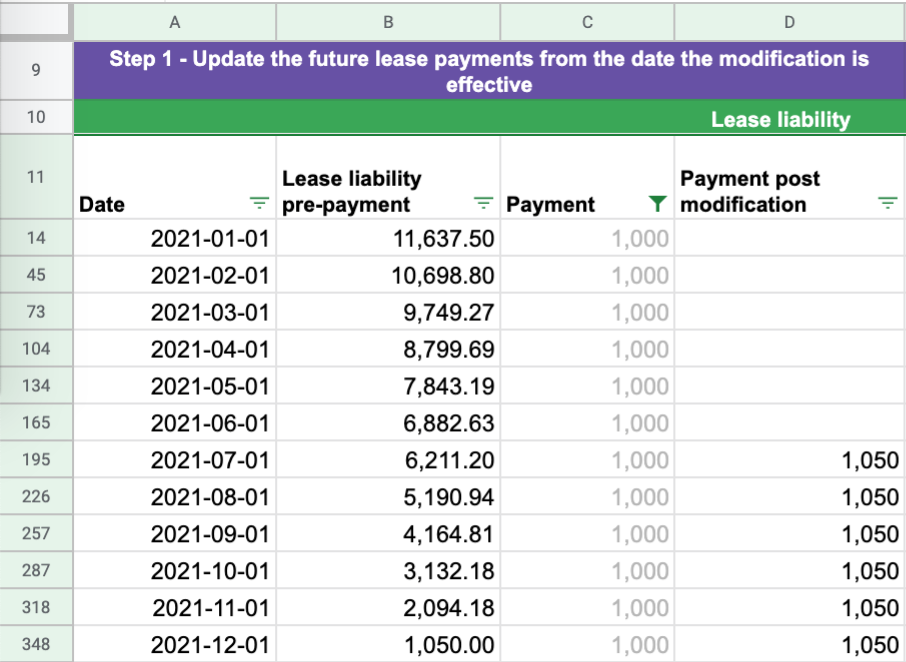When a modification occurs, an additional column is required to calculate the updated future lease payments. If you were to update the original column, it would then change the initial net present value calculations. This is something you do not want. For Example 2, the lessor communicated the revised payment amount on June 15 that fixed payments will be increased by 5% from July 1 from \$1,000 to \$1,050.

### Step 2 - Determine the appropriate discount rate and re-calculate the lease liability on the date effective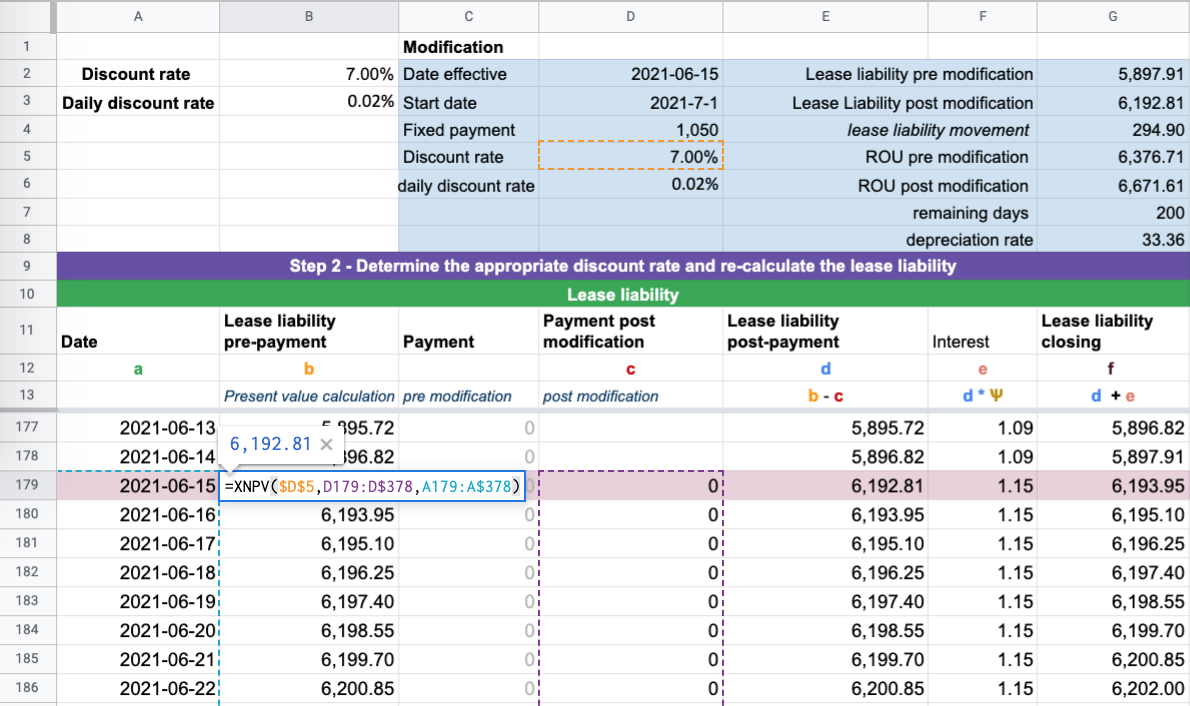This is where a solid understanding of modification accounting is required. There are two critical components to this calculation:

1. The remeasurement of the lease liability occurs on 2021-06-15. That’s because that is when the lessee is made aware of the change in the future lease payments. Because of this change, the lessee has a larger lease liability because of the increase in the future lease payments.
2. In this example, the increase in fixed payments is a result of a CPI increase. As a result, there’s no need to update the discount rate.

This treatment is stated in paragraph IFRS 16.42(b):

If there is a change in future lease payments resulting from a change in an index or a rate used to determine those payments, including for example a change to reflect changes in market rental rates following a market rent review. The lessee shall remeasure the lease liability to reflect those revised lease payments only when there is a change in the cash flows (ie when the adjustment to the lease payments takes effect). A lessee shall determine the revised lease payments for the remainder of the lease term based on the revised contractual payments.

And IFRS 16.43

In applying paragraph 42, a lessee shall use an unchanged discount rate, unless the change in lease payments results from a change in floating interest rates. In that case, the lessee shall use a revised discount rate that reflects changes in the interest rate.

A typical example of a floating rate the lease accounting standard is referring to is LIBOR. In this case of a CPI review, there’s no need to reassess the discount rate. If there’s an increase in the fixed payments due to a contractual change, that will result in applying an updated discount rate.

In reference to Example 2, you can see that the XNPV present value calculation has been updated to reflect the CPI increase in the lease payments. In doing so, this changes the value of the lease liability.

a) Lease liability post payment will subtract payments from column D as opposed to column C: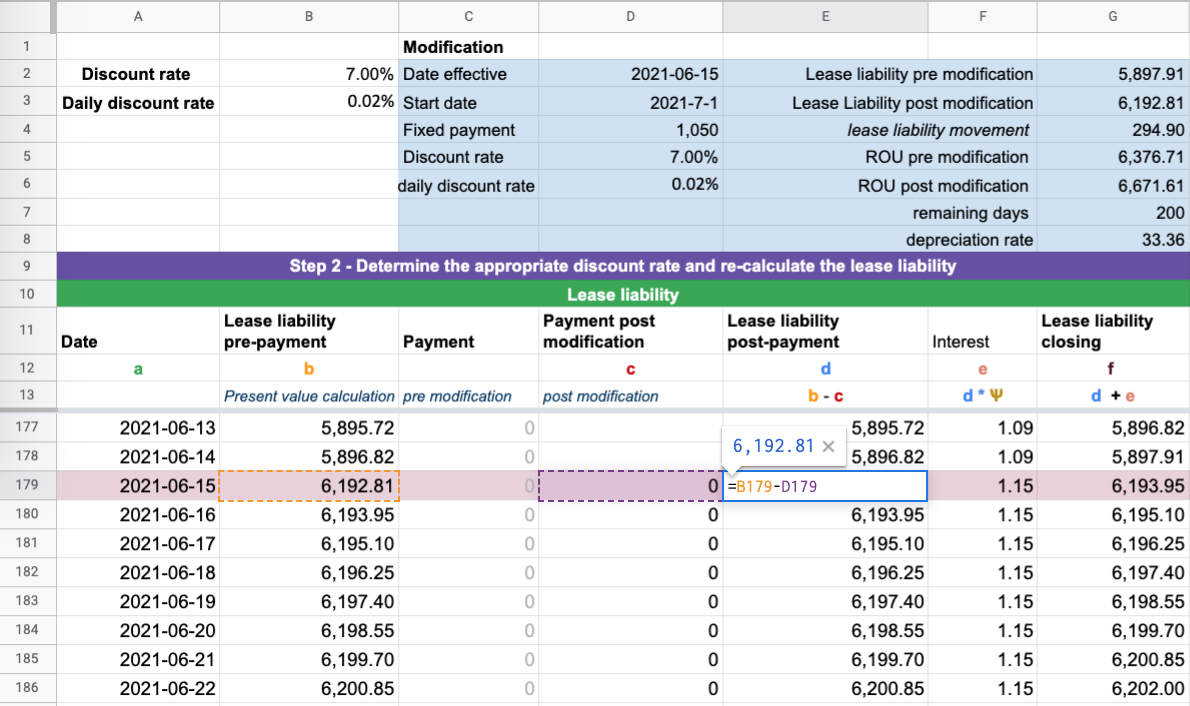b) Daily interest calculation will use the updated daily discount rate (in this example there is no change):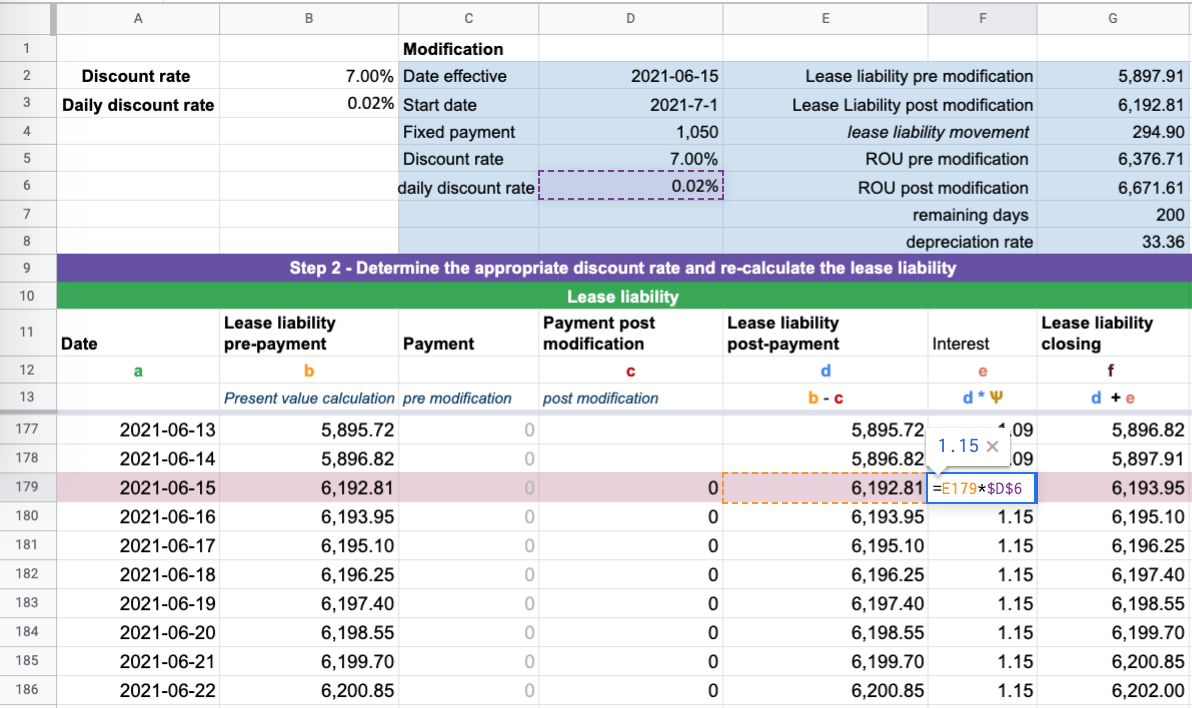Once you have made the following changes, ensure that the modified lease liability unwinds to \$0 based on the updated inputs.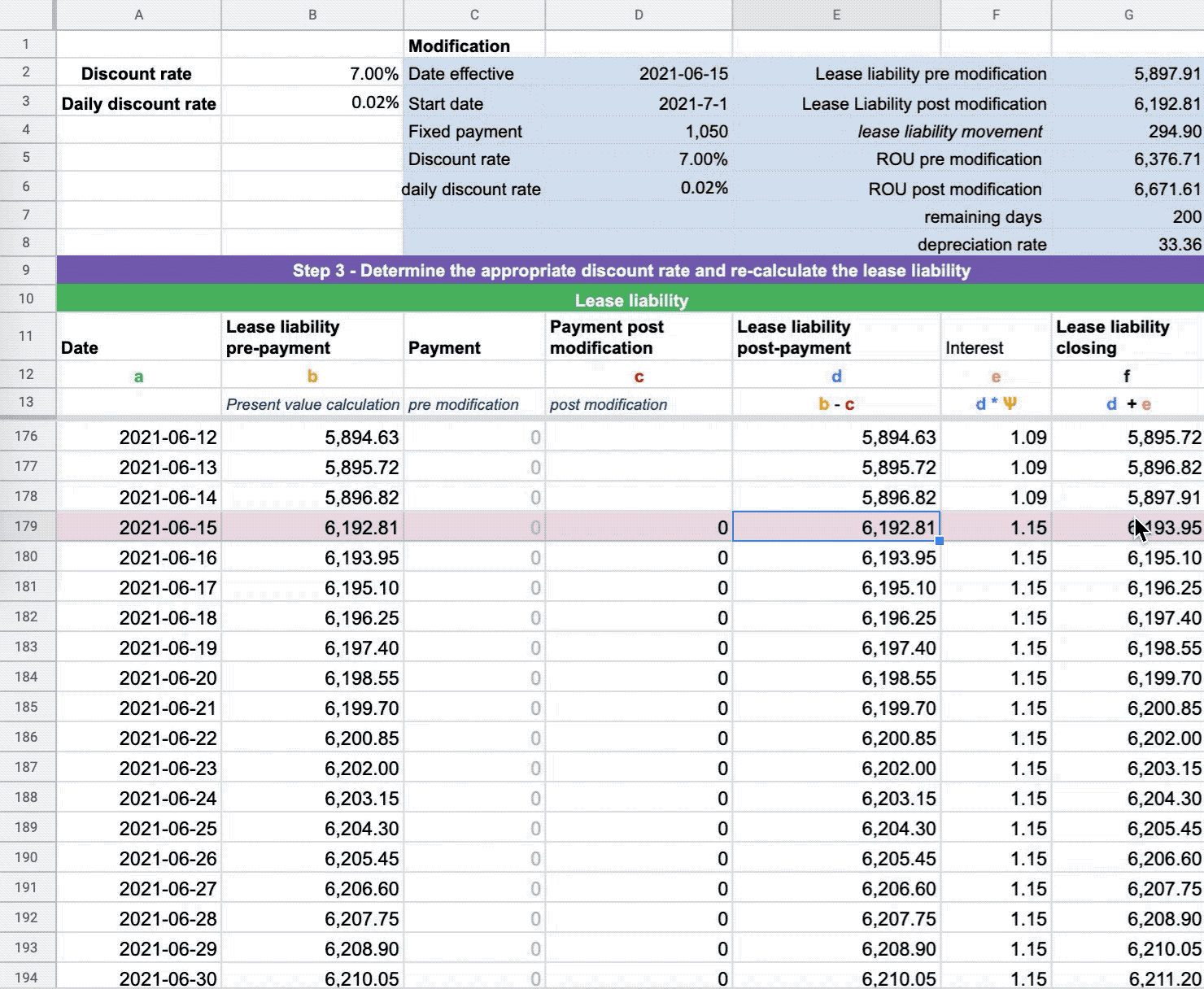If the lease liability balance does not go to zero it’s a result of not making one of the above updates. Don’t forget the updates need to be applied to each row of the calculation.

### Step 3 - Update the right of use asset value based on the modification amount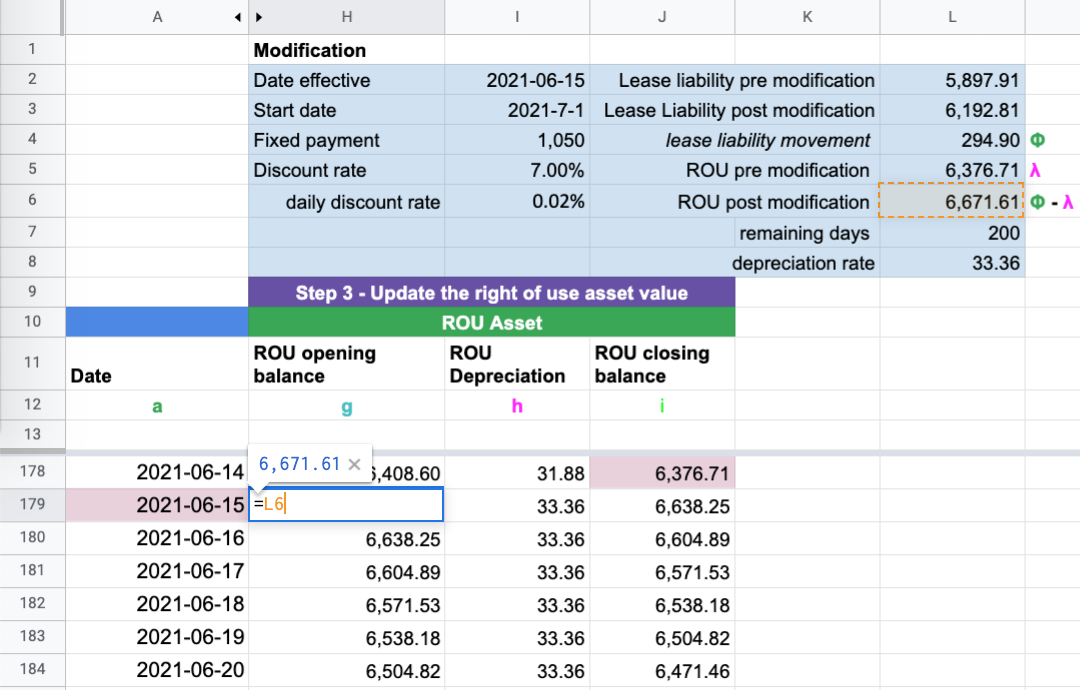The first step is to work out the movement of lease liability pre and post-modification. With Example 2, the lease liability amount increased by \$294.90. The lease liability pre-modification was \$5,897.91. Post modification, the lease liability value is \$6,192.81.

Now the journals, to increase the lease liability, that's a credit. So, where does the debit go?

The debit side goes to the right of use asset:

Dr Right of use asset \$294.90

Cr Lease liability \$294.90

Because of the above journal entry, the right of use asset on 2021-06-15 pre-modification was \$6,376.71 post modification, \$6,671.61.

### Step 4 - Update the depreciation rate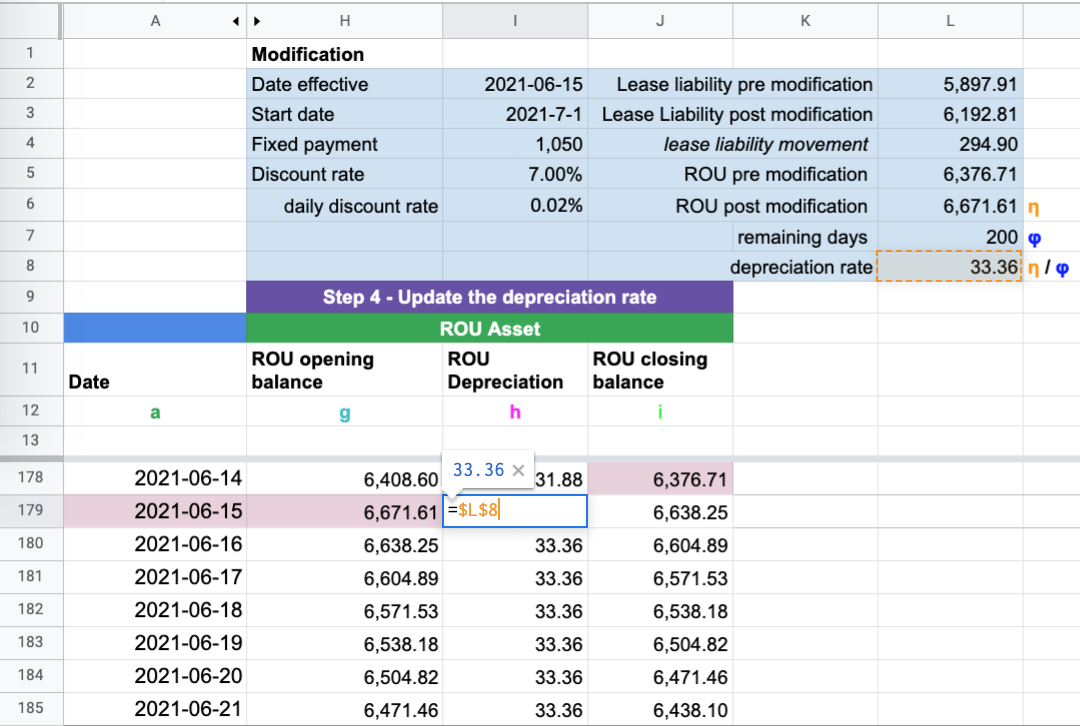Because the value of the right of use asset has changed, the depreciation rate must also be updated. If this is not done, the right of use asset will not depreciate to zero.

To calculate this amount, use the post modification value of the right of use asset value and divide that number by the remaining days of the useful life of the ROU.

In reference to Example 2 that is:

• 6,671.61 (post modification right use asset value)/200 (number of days remaining of right of use assets useful life = \$33.36

The last check is to make sure the right of use asset depreciates to zero: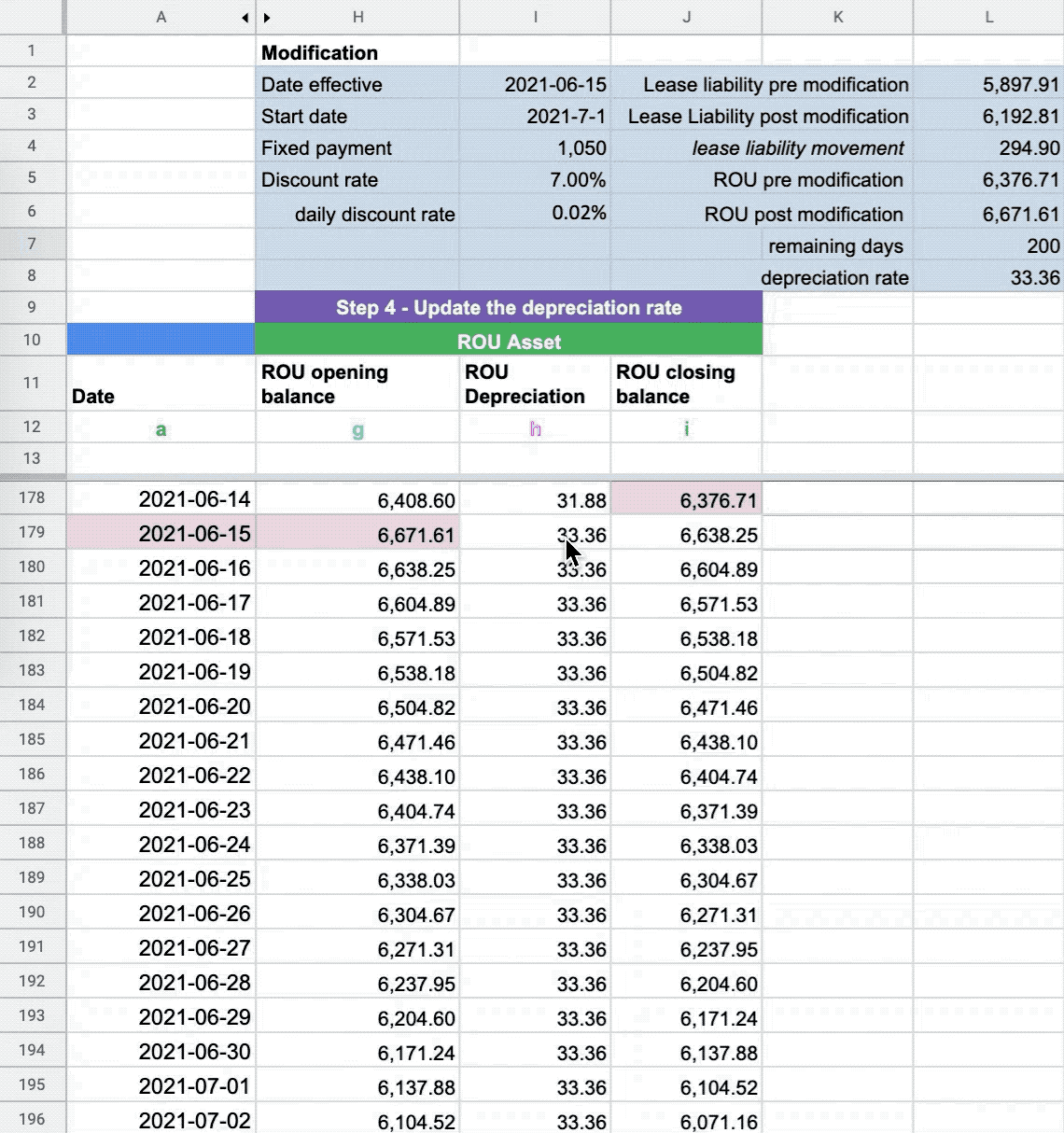## Conclusion

With that last check, you're done! Some other articles you might find helpful are:

Now I can personally attest when doing the calculations for this article. I made a few blunders. How can I fix this? There's an inherent risk of manual error doing anything manually. The alternative is to automate these calculations by using lease accounting software like Cradle.

## Try Cradle free for 30 days.

Starting at only \$99/month. Automate your lease accounting calculations, journal entries, and financial reports by simply entering the contractual details of the lease.

Start Free Trial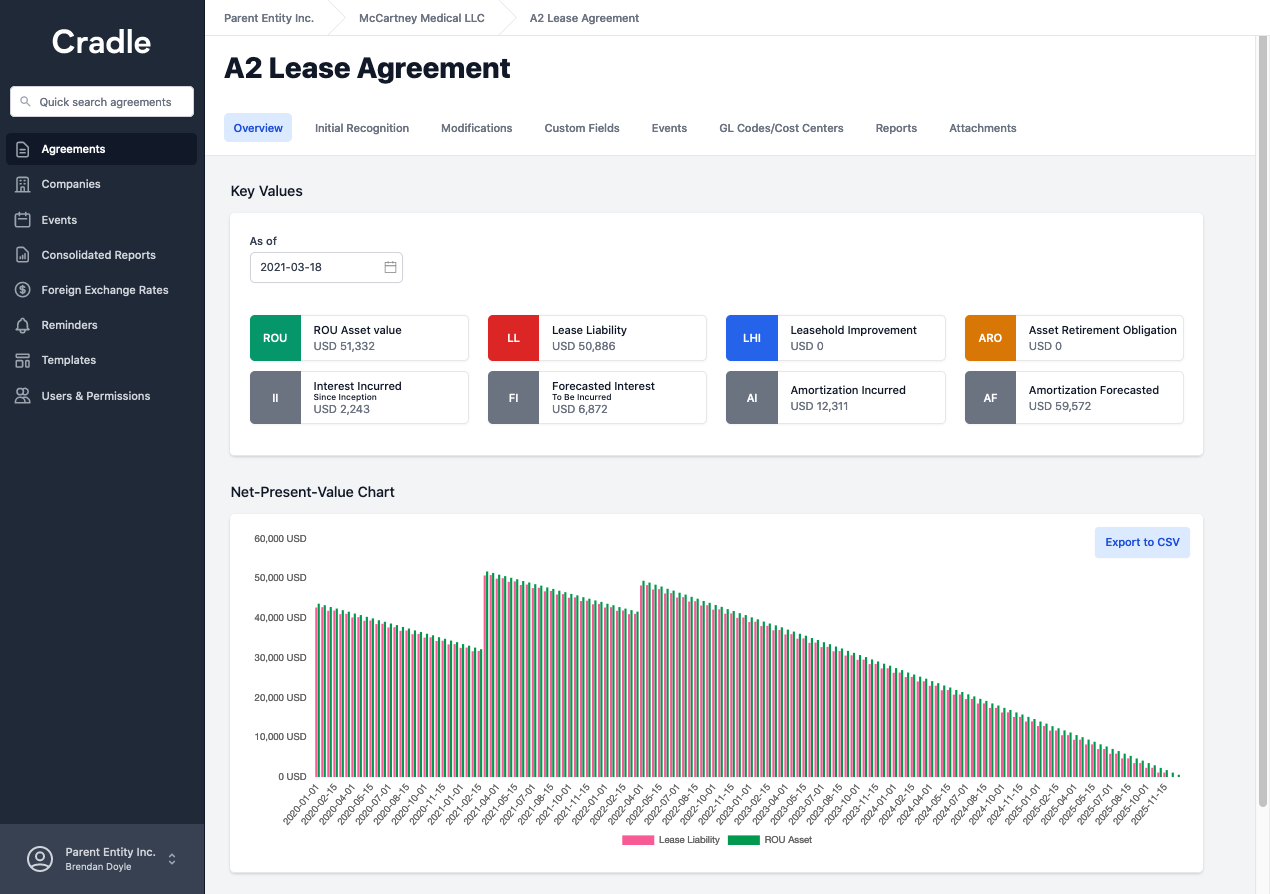## FooterHere at Cradle, our mission is simple; it's at the foundation of everything that we do. We want to make accountants' lives easier by leveraging technology to free up their time to focus on running the business.

140 Yonge St.
Suite 200
Toronto, ON M5C 1X6

US
Suite #73591
Torrance, CA 90503
USA

### Legal